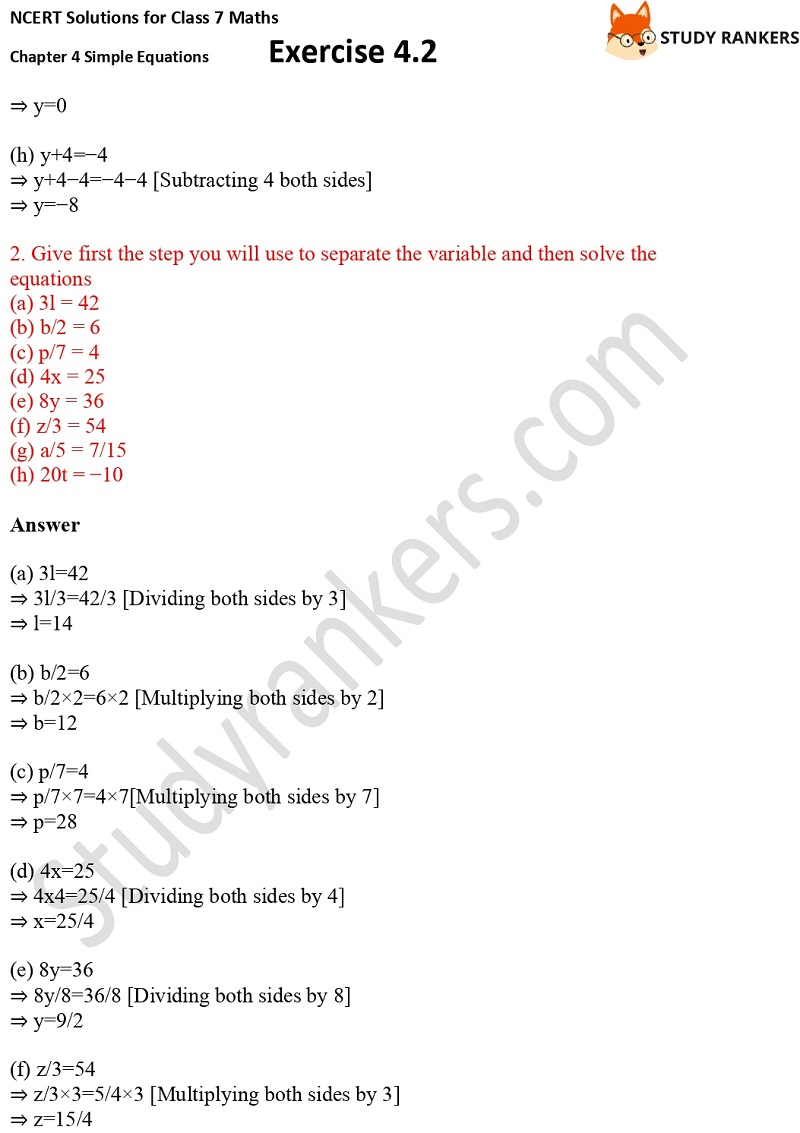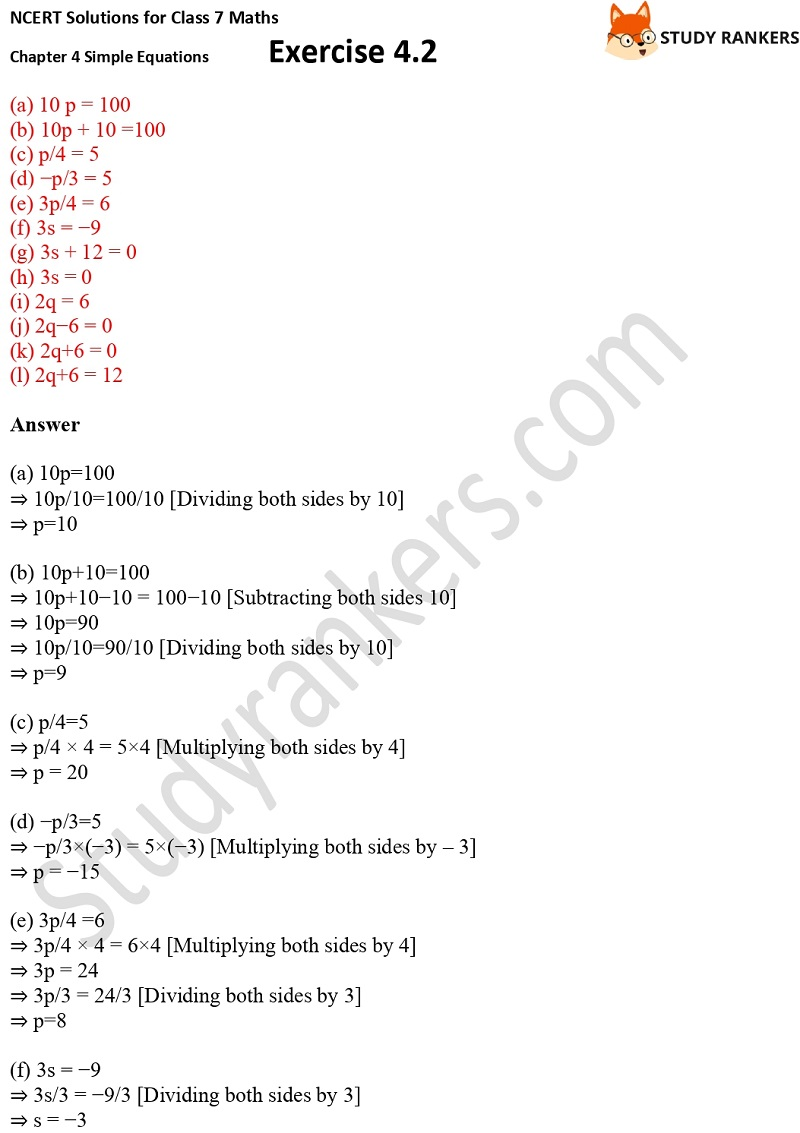>

# NCERT Solutions for Class 7 Maths Ch 4 Simple Equations Exercise 4.2

In this page, you will find Chapter 4 Simple Equations Exercise 4.2 NCERT Solutions for Class 7 Maths which can be useful in completing your homework on time. It is useful in understanding the basic concepts of the chapter properly and solving your doubts. These NCERT Solutions for Class 7 are detailed and accurate through which you can clear your doubts.

In Exercise 4.2, you have to give first the step you will use to separate the variable and then solve the equations.X